# Fractions + direct relationship - math problems

#### Number of problems found: 72

• Snack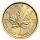CZK 450 was paid for a snack for 30 pupils. How many CZK will we pay for the same snack for 28 pupils?
• Scale of the mapDetermine the map's scale, which is the actual distance of 120 km l represented by a segment long 6 cm.
• PediatricianPediatrician this month of 20 working days takes 8 days holidays. What is the probability that on Monday it will be at work?
• Three 43Three brothers inherited a cash amount of 62,000, and they divided it among themselves in the ratio of 5:4:1. How much more is the largest share than the smallest share?
• In theIn the national park, the ratio of the wooded area to grassland is 4: 1. The total area is 385km2. What area is wooded?
• Jan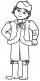Jan is 13 years and Peter 21 years old. For how many years will their ages in the ratio of 7:9?
• Pumps 5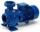5 pump pumped in 3 hours 1800 hl of water. How many hectoliters of water pumped 4 equally powerful pumps in 6 hours?
• In the bakery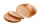In 2.25 hours, 24 pieces of bread are baked in the bakery. How many breads are baked in the bakery in 6 hours and 45 minutes?
• Scale of the map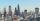The distance between two cities is actually 30 km and the map is 6 cm. What is the scale of the map?
• PamelaLara Pamela spends her salary of 3000 for food, clothing, recreation and savings, which are in the ratio of 48:20:15:37, respectively. How much does he spend for savings?
• Cows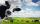4 cows spent 16 bags of hay in 5 days. How many bags of hay sacks are needed for 5 cows for seven days?
• Divers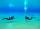20-member group of divers carries the air for 15 hours of diving. 4 members of the expedition sick or wasn't came. How many hours is sufficient air to other divers?
• Proportion 3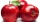For every 8 mango trees in the orchard, there are 4-star apple trees. If there are 1320 trees, how many trees of each kind are there?
• Babysitting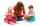The amount that Susan charges per hour for babysitting are directly proportional to the number of children she is watching. She charges \$4.50 for 3 kids. How much would she charge to babysit 5 kids?
• Proportion 2A car is able to travel 210 km in 3 hours. How far can it travel in 5 hours? Put what kind of proportion is this and show your solution.
• Points on line segmentPoints P & Q belong to segment AB. If AB=a, AP = 2PQ = 2QB, find the distance: between point A and the midpoint of the segment QB.
• GardenThe rectangular garden has dimensions of 27 m and 30 m. Peter and Katka split it in a ratio of 4:5. How many square meters did Katkin measure part of the garden?
• Milk2cheese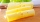From 40 liters of milk is produced 8 kg of cheese how many liters of milk are needed to produce 2 kg of cheese?
• Five combers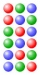Five combers harvest 12 rows of strawberries in 4 hours. How many rows of strawberries will two combers harvest in 10 hours?
• Unknown number 5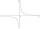Daniel thinks an integer. When he change this number at a ratio of 2:5, he got the number 2.8. Determine what number think Daniel.

Do you have an interesting mathematical word problem that you can't solve it? Submit a math problem, and we can try to solve it.

We will send a solution to your e-mail address. Solved examples are also published here. Please enter the e-mail correctly and check whether you don't have a full mailbox.

Please do not submit problems from current active competitions such as Mathematical Olympiad, correspondence seminars etc...

Need help to calculate sum, simplify or multiply fractions? Try our fraction calculator. Fraction Word Problems. Direct relationship - math problems.